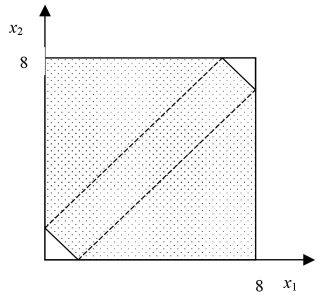# Convex Hull Relaxation for a linear disjunction

Suppose the following disjunction with i terms: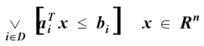(1)

The convex hull relaxation for the previous disjunction can be defined as follows: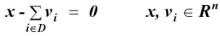(2)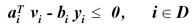(3)(4)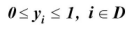(5)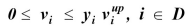(6)

Where:
(2) Corresponds to the continuous variables disaggregation,
(3) Are the original constraints of each disjunction term where continuous variables are replaced by the disaggregated variables,
(4) Only one disjunction term must be true,
(5) Bounds on the discrete variables y,
(6) Bounds on the dissagregated variables.

Example:
Having the same example as the BigM relaxation:(7)

The feasible region of this disjunction is: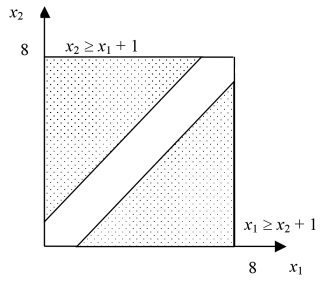The equations for the convex hull are as follows: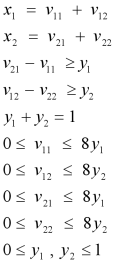The feasible region of the convex hull relaxation is as follows: